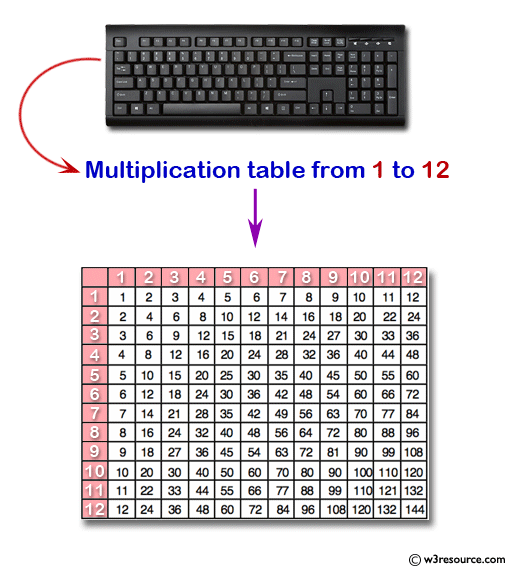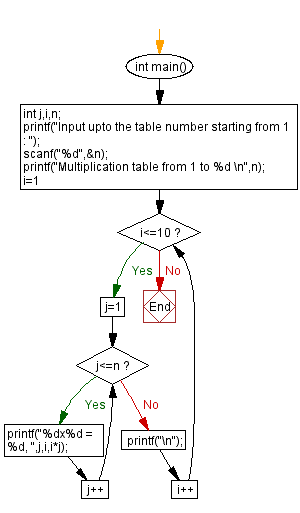﻿ C : Display n number of multiplication table vertically# C Exercises: Display n number of multiplication table vertically

## C For Loop: Exercise-7 with Solution

Write a program in C to display the multiplication table vertically from 1 to n.

Pictorial Presentation:Sample Solution:

C Code:

``````#include <stdio.h>
void main()
{
int j,i,n;
printf("Input upto the table number starting from 1 : ");
scanf("%d",&n);
printf("Multiplication table from 1 to %d \n",n);
for(i=1;i<=10;i++)
{
for(j=1;j<=n;j++)
{
if (j<=n-1)
printf("%dx%d = %d, ",j,i,i*j);
else
printf("%dx%d = %d",j,i,i*j);

}
printf("\n");
}
}
```
```

Sample Output:

```Input upto the table number starting from 1 : 8
Multiplication table from 1 to 8
1x1 = 1, 2x1 = 2, 3x1 = 3, 4x1 = 4, 5x1 = 5, 6x1 = 6, 7x1 = 7, 8x1 = 8
1x2 = 2, 2x2 = 4, 3x2 = 6, 4x2 = 8, 5x2 = 10, 6x2 = 12, 7x2 = 14, 8x2 = 16
1x3 = 3, 2x3 = 6, 3x3 = 9, 4x3 = 12, 5x3 = 15, 6x3 = 18, 7x3 = 21, 8x3 = 24
1x4 = 4, 2x4 = 8, 3x4 = 12, 4x4 = 16, 5x4 = 20, 6x4 = 24, 7x4 = 28, 8x4 = 32
1x5 = 5, 2x5 = 10, 3x5 = 15, 4x5 = 20, 5x5 = 25, 6x5 = 30, 7x5 = 35, 8x5 = 40
1x6 = 6, 2x6 = 12, 3x6 = 18, 4x6 = 24, 5x6 = 30, 6x6 = 36, 7x6 = 42, 8x6 = 48
1x7 = 7, 2x7 = 14, 3x7 = 21, 4x7 = 28, 5x7 = 35, 6x7 = 42, 7x7 = 49, 8x7 = 56
1x8 = 8, 2x8 = 16, 3x8 = 24, 4x8 = 32, 5x8 = 40, 6x8 = 48, 7x8 = 56, 8x8 = 64
1x9 = 9, 2x9 = 18, 3x9 = 27, 4x9 = 36, 5x9 = 45, 6x9 = 54, 7x9 = 63, 8x9 = 72
1x10 = 10, 2x10 = 20, 3x10 = 30, 4x10 = 40, 5x10 = 50, 6x10 = 60, 7x10 = 70, 8x10 = 80
```

Flowchart:C Programming Code Editor:

Improve this sample solution and post your code through Disqus.

What is the difficulty level of this exercise?

Test your Programming skills with w3resource's quiz.

﻿